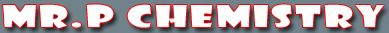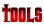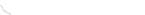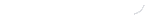activity seriesbond energybond lengthscalculatorclockcommon namesconversion factors: energyconversion factors: lengthconversion factors: massconversion factors: pressureconversion factors: temp.conversion factors: volumecovalent prefixesdensity solverdiatomic elementse-config. chartelement listgas law formulasKa's of polyprotic acidsKa's of weak acidsKb's of weak basesmetric conversion chartmole conversion chartmolecular geometriesorganic prefixesperiodic tableperiodic table (flash)pH/pOH converterpolyatomic ionspressure converterSI unitssolubility chartsolubility of salts rulessolubility product constantsstoichiometry charttemp. conversiontemp. formulasthermodynamic datavapor pressure of water